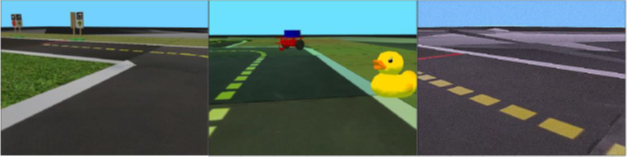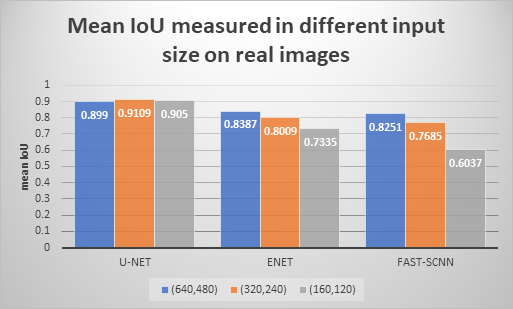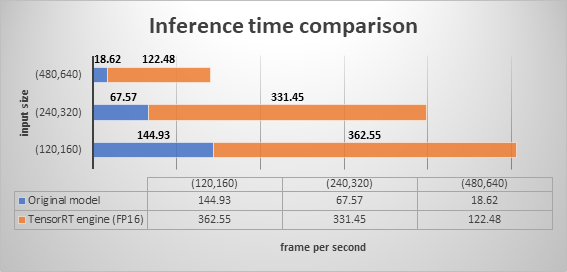Deep Learning-based Semantic Segmentation in Simulation and Real-World for Autonomous Vehicles — part 2

Author: Zsombor Tóth

Part 1 of this blog post can be found here.

Introduction

Deep Learning is one of the most important techniques of autonomous vehicles nowadays. There are many components of a self-driving vehicle that can be realized with the help of deep neural networks (e.g. car and pedestrian detection, depth estimation, scene interpretation, controlling, etc.). Among the many, semantic segmentation is one of the most essential components without a doubt. There have already been a number of works on semantic segmentation, however, it is still challenging to develop high accuracy solutions in application domains where only a few, or no precise pixel-wise class labels are available.

During my work, I created a unique solution for collecting segmented data from the Duckietown simulator to create my own synthetic dataset for training. I extended this training dataset with domain randomization and augmentation techniques in order to make the models more adaptable to the real environment. I finetuned my models with only 70 hand-segmented real images, as a result of which I achieved further accuracy improvements. Further on, I made the U-Net model more efficient using a complex optimization procedure called TensorRT.

Improving training dataset

I trained with simulation data oversampled with domain randomization and augmentation techniques. The number of synthetic images used for training is 30.000 (~ 25Gb) and the number of validation samples is 2.000. Domain randomization is a targeted technique for making modifications to simulated images that allow us to better adapt our model to reality. We call this research problem transfer learning when we want to transfer a model trained for a given task to a similar problem. In my case, to adapt from simulation to real environment. The hypothesis of the method is as follows: if the variability in simulation is significant enough, models trained in the simulation will generalize to the real world. During domain randomization, many variabilities can be introduced, for example:

• randomly generated distractor objects with different shape and texture

• not realistic textures of the observed objects

• different orientations, brightness, and properties of the lights

• different position, orientation, and field of view of the camera

• random RGB values

These domain randomized formulas can be implemented in the simulation, which is supported by the Duckietown simulator, so these instructions can be specified as parameters.

I used augmentation methods to further expand the dataset. To implement this, I used the imgaug library, which supports several augmentation techniques. The library supports the simultaneous transformation of the segmentation map and the original image. The transformations I used are the following: horizontal flip, gaussian blur, contrast, gaussian noise, color brightness, affine transformations and sharpen. Figure 1 shows a domain randomized and an augmented image.Fig. 1: On the left is an original simulation image, in the middle is a domain randomized image, and on the right is an augmented image.

Results

I separated the training process into 2 major parts based on what type (simulation or real) samples I used for training. On this basis, in the first part, I used 30.000 training samples and 2.000 validation samples generated by the simulator and oversampled with domain randomization techniques and augmentation techniques. In the second part, I used the weights of the models created in the first part as pretrained weights and I finetuned the models with a smaller real data set (70 images).

I did not apply augmentation technologies to the real images, nor did I mix synthetic samples between the training data. As a result, the mean IoU values measured on the simulated data have decreased, which is not a big problem in the current use case, since the goal is segmentation with the neural network on real data. If we also want to use the models in the simulator, it would be more practical to use a mixed training dataset, which contains both real and simulated data. The following diagram illustrates the results of the finetuning. Based on the diagram, I reached the highest value for the U-Net medium input size (320x240) with a mean IoU value of 0.91.Fig. 2: The result of finetuning for each model. Each column represents the mean IoU values measured on real images for different input image sizes.

With the help of TensorRT, which is a high-performance library developed by Nvidia for accelerating deep learning inference, I achieved a significant increase in inference time. The inference time values are shown in the following figure.Fig. 3: Comparison of U-Net model inference time for original and TensorRT optimized engine. Each column represents the values measured for different input image sizes, while each value indicates the number of frames processed per second.

In Fig. 3, we can observe that I achieved a significant increase in inference time. The value measured is 6.5 times higher for the largest input size, nearly 5 times higher for the medium input size, and 2.5 times better for the smallest input size. Comparing this observation with the mean IoU values shown in Fig. 2, we conclude that the best result in terms of speed and accuracy can be obtained with the medium input-sized model. This trained model can even be used for real-time processing on an edge device, for example on a Duckiebot controlled with Jetson Nano.

The semantic segmentation capability of the final model is shown in the following animation.